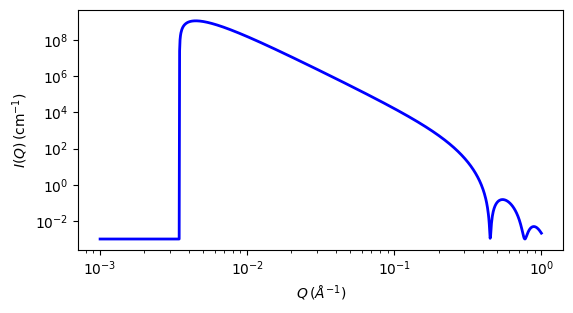# surface_fractal

Fractal-like aggregates based on the Mildner reference

Parameter

Description

Units

Default value

scale

Scale factor or Volume fraction

None

1

background

Source background

cm-1

0.001

10

fractal_dim_surf

Surface fractal dimension

None

2

cutoff_length

Cut-off Length

500

The returned value is scaled to units of cm-1 sr-1, absolute scale.

This model calculates the scattering from fractal-like aggregates based on the Mildner reference.

Definition

The scattering intensity $$I(q)$$ is calculated as

\begin{align*} I(q) &= \text{scale} \times P(q)S(q) + \text{background} \\ P(q) &= F(qR)^2 \\ F(x) &= \frac{3\left[\sin(x)-x\cos(x)\right]}{x^3} \\ S(q) &= \Gamma(5-D_S)\xi^{\,5-D_S}\left[1+(q\xi)^2 \right]^{-(5-D_S)/2} \sin\left[-(5-D_S) \tan^{-1}(q\xi) \right] q^{-1} \\ \text{scale} &= \text{scale factor}\, N V^1(\rho_\text{particle} - \rho_\text{solvent})^2 \\ V &= \frac{4}{3}\pi R^3 \end{align*}

where $$R$$ is the radius of the building block, $$D_S$$ is the surface fractal dimension, $$\xi$$ is the cut-off length, $$\rho_\text{solvent}$$ is the scattering length density of the solvent and $$\rho_\text{particle}$$ is the scattering length density of particles.

Note

The surface fractal dimension is only valid if $$1<D_S<3$$. The result is only valid over a limited $$q$$ range, $$\tfrac{5}{3-D_S}\xi^{\,-1} < q < R^{-1}$$. See the reference for details.Fig. 121 1D plot corresponding to the default parameters of the model.

Source

surface_fractal.py $$\ \star\$$ surface_fractal.c $$\ \star\$$ sas_gamma.c $$\ \star\$$ sas_3j1x_x.c

References

1. D Mildner and P Hall, J. Phys. D: Appl. Phys., 19 (1986) 1535-1545

Authorship and Verification

• Author: